Electron. J. Differential Equations, Vol. 2020 (2020), No. 60, pp. 1-15.

### Existence and multiplicity for a superlinear elliptic problem under a non-quadradicity condition at infinity Leandro Recova, Adolfo Rumbos

Abstract:
In this article, we study the existence and multiplicity of solutions of the boundary-value problem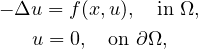where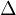denotes the N-dimensional Laplacian,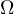is a bounded domain with smooth boundary,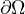, in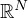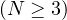, and f is a continuous function having subcritical growth in the second variable. Using infinite-dimensional Morse theory, we extended the results of Furtado and Silva  by proving the existence of a second nontrivial solution under a non-quadradicity condition at infinity on the non-linearity. Assuming more regularity on the non-linearity f, we are able to prove the existence of at least three nontrivial solutions.

Submitted February 28, 2020. Published June 16, 2020.
Math Subject Classifications: 35J20.
Key Words: Semilinear elliptic boundary value problem; superlinear subcritical growth; infinite dimensional Morse theory; critical groups.

Show me the PDF file (361 KB), TEX file for this article.Leandro L. Recôva T-Mobile Inc. Ontario, CA 91761, USA email: leandro.recova3@t-mobile.com Adolfo J. Rumbos Department of Mathematics Pomona College Claremont, CA 91711, USA email: arumbos@pomona.edu

Return to the EJDE web page